Subsections

## 3.4.3 Nanodot Modeling Using the MC Method

The simulator is implemented by first calculating the shape of the nanodot or nanowire with the previously mentioned empirical equations which depend on the oxidation time, applied voltage, ambient humidity, and nanowire orientation. Afterwards, a given number of particles is distributed above the silicon surface, their position following the pattern of the desired surface deformation. Finally, each particle is accelerated towards the surface, causing it to collide with the wafer. Upon impact, the silicon dioxide is advanced deeper into the silicon, while it simultaneously grows into the ambient. The result is an oxide nanodot or nanowire having the desired height and width, depending on the processing variables of voltage, time, humidity, and orientation. The method of imprinting a desired particle distribution onto a wafer surface is represented graphically in Figure 3.19.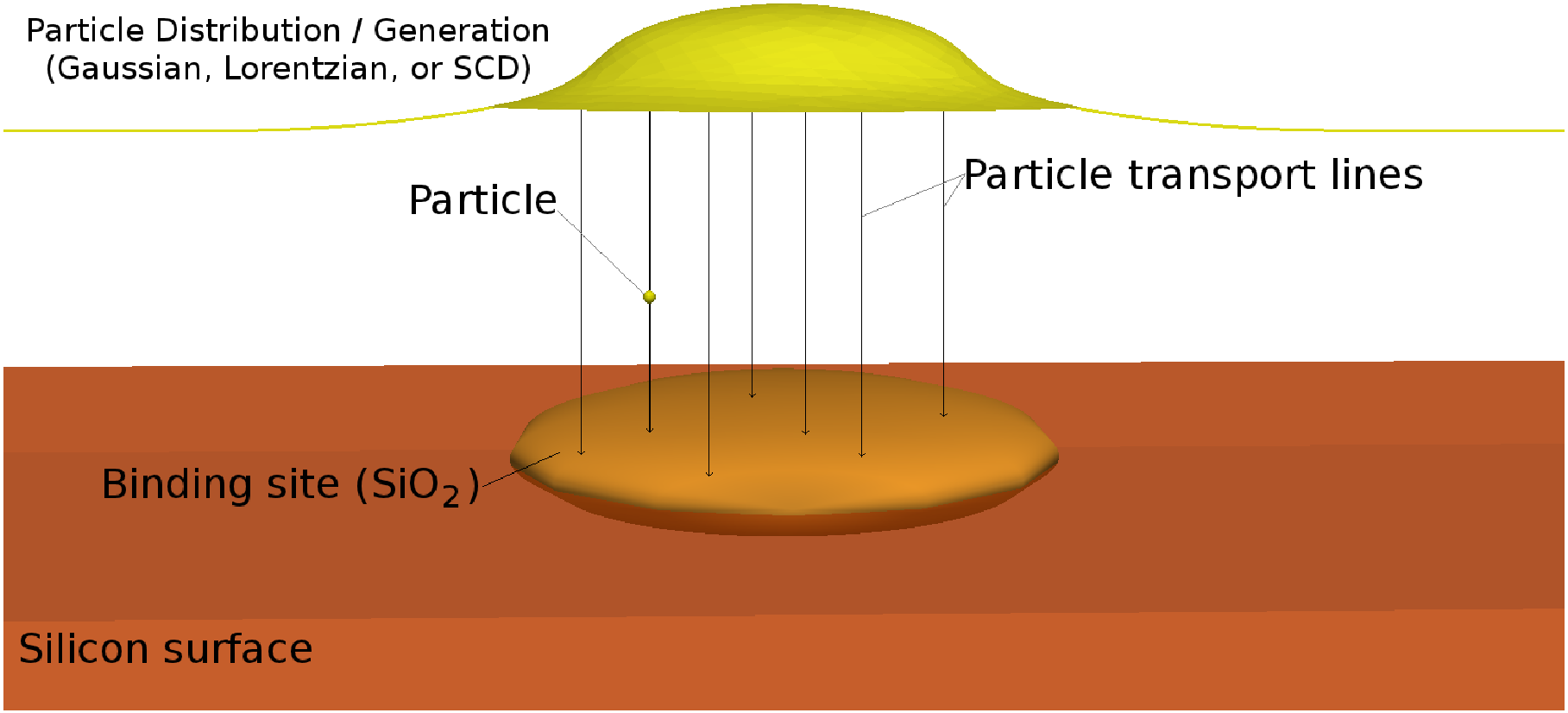A flow chart summarizing the simulation steps is given in Figure 3.20, which describes how the desired particle distribution is effectively imprinted onto the LS surface using the MC method.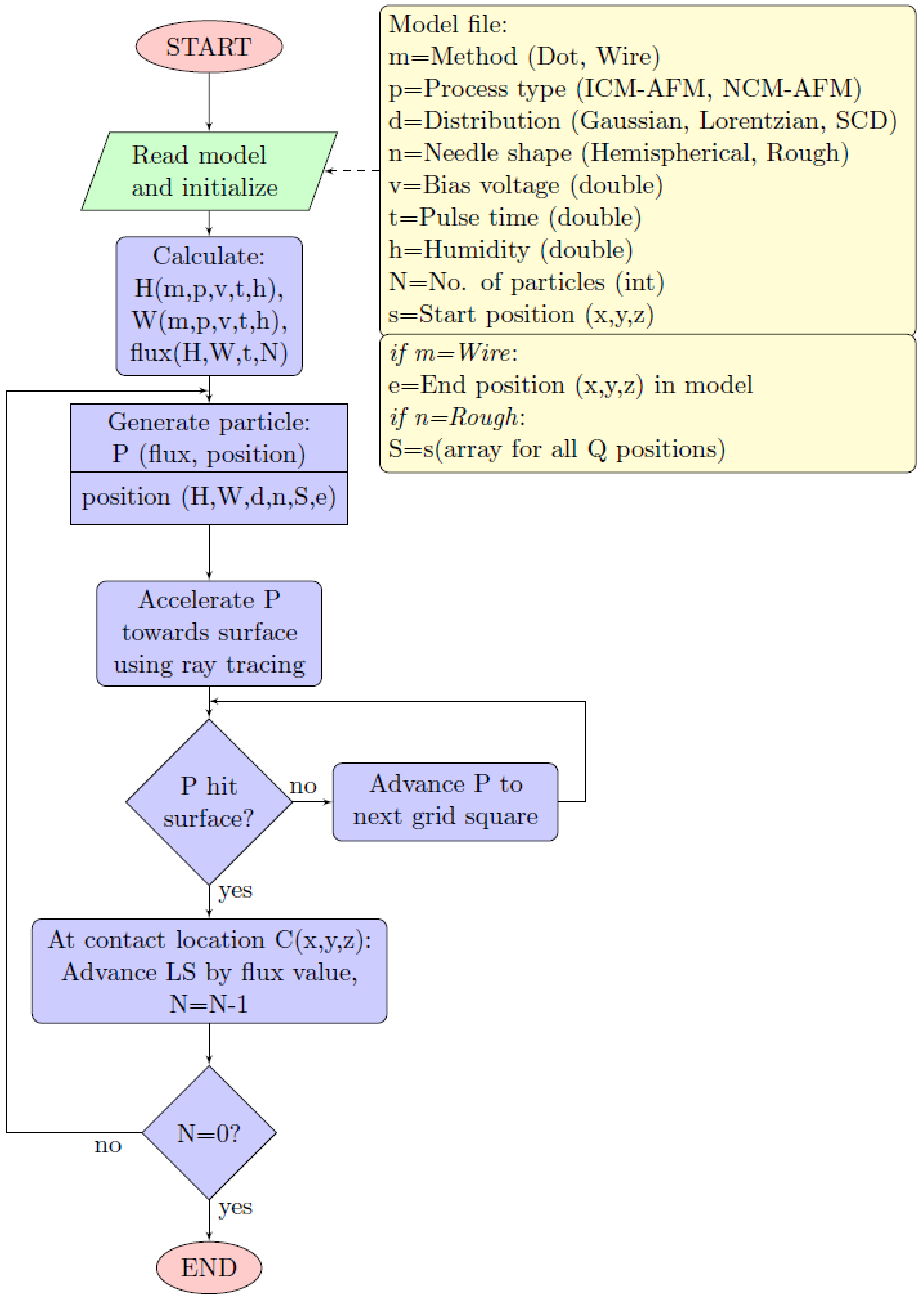### 3.4.3.1 Gaussian Particle Distribution

As seen from the previous discussion regarding the MC model for AFM oxidation from Figure 3.20, a method to distribute particles according to a desired distribution is required. Some literature approximate the final oxide dot topography with a Gaussian curvature , while some suggest a Lorentzian profile . The quantile function of the one-dimensional Gaussian distribution, required for the generation of a random particle position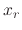, is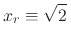erf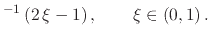(123)

Because of the error function, the quantile Gaussian function is not easily implementable with a random distribution in the MC environment and hence another model is desired. The model implemented in the simulator is based on the well known Marsaglia polar method . This method suggests a way to generate two independent standard normal random variables. The first step is the generation of an evenly distributed random location (r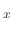, r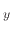) within a circle of unity radius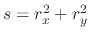, where rand rare evenly distributed random numbers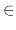(-1, 1). The Gaussian distributed coordinates (x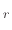, y) can then be calculated using the Marsaglia equations(124)

A sample Gaussian distributed nanodot is shown in Figure 3.21.

Figure 3.21: Nanodot generated using Gaussian particle distribution. The vertical dimension has been scaled by 20 for better visualization. (a) NCM nanodot generated using a Gaussian distribution of particles and (b) Diagonal cross-section of the nanodot from Figure 6.13a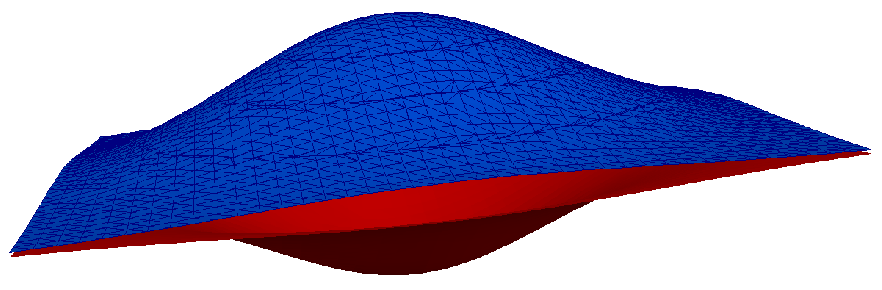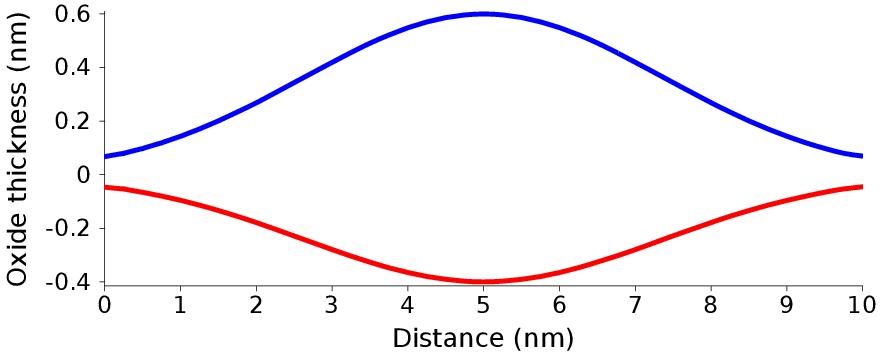(a) NCM Gaussian nanodot (b) Cross-section.

The Gaussian distribution is well known; however from Figure 3.22, it is suggested that a Lorentzian distribution is a better fit to the final shape of the desired nanostructure .

Figure 3.22: (a) Comparison between the Gaussian distribution and the surface charge density and (b) comparison between the Lorentzian distribution and the surface charge density.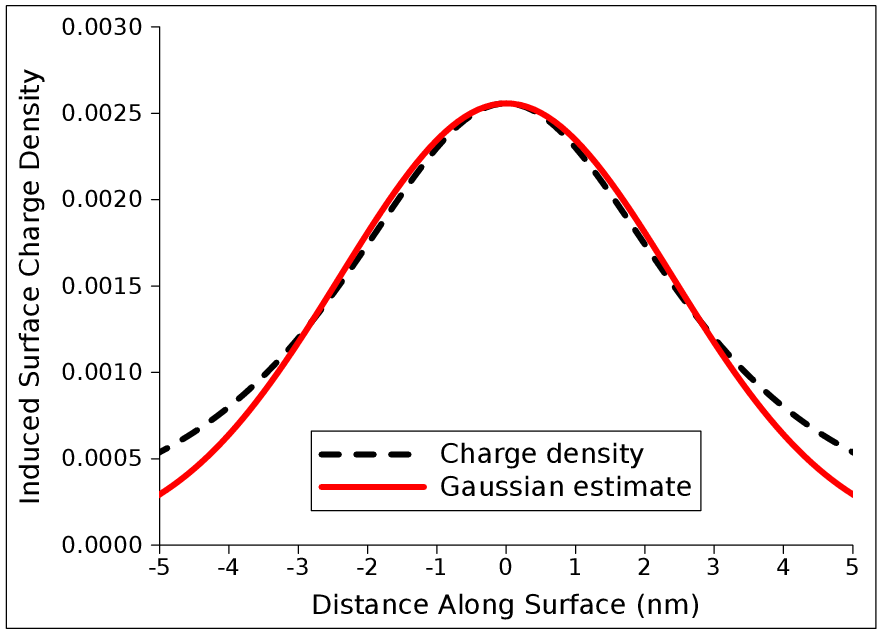(a) Gaussian distribution. (b) Lorentzian distribution.

The implementation of the Gaussian distribution was performed successfully, while a similar approach to the Lorentzian distribution was attempted without much success. Therefore, in order to generate particles according to a Lorentzian distribution, a novel technique for a particle distribution which follows the Lorentzian equation is developed. The technique results in equations similar to the Marsaglia-Polar equations from (3.53).

### 3.4.3.2 Lorentzian Particle Distribution

One-Dimensional Distribution

The normalized Probability Density Function (PDF) of the Lorentzian distribution is given by: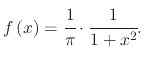(125)

The Cumulative Probability Density (CPD) is found by integrating the PDF to obtain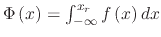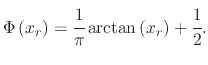(126)

The quantile function of the Lorentzian distribution, required for particle generation, is the inverse CPD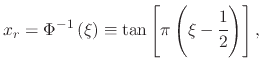(127)

where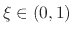is a uniformly distributed random number. Therefore, using (3.56), a random particle can be generated to follow the Lorenzian distribution by first generating an evenly distributed value for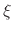.

Two-Dimensional Distribution

The same analysis shown for the one-dimensional Lorentzian distribution must be performed in order to generate a two-dimensional Lorentzian quantile function. The two-dimensional Lorenzian distribution is required when a three-dimensional nanodot needs to be simulated.

The PDF of the two-dimensional Lorentzian distribution can be represented as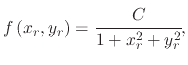(128)

where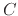is the normalization constant. Using polar coordinates, where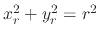and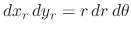, it can easily be shown that the PDF cannot be normalized in the entire real space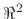. We therefore must normalize the equation to a desired maximum radius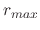.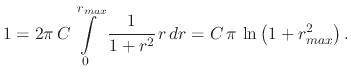(129)

The normalization constant follows from the CPD, normalized to(130)

The CPD for the two-dimensional distribution, normalized tocan then be written as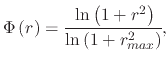(131)

where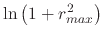is treated as a-dependent constant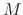. By inverting the CPD and solving for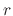the two-dimensional Lorentzian quantile function is found, which is required for particle generation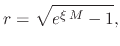(132)

whereis a uniformly distributed random number. Using (3.61), a random particle location can be generated in two-dimensional space to follow the Lorenzian distribution. The generated location is a radial distance from the center of the distribution.

An evenly distributed anglebetween 0 and 2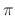is generated and the final particle position is given by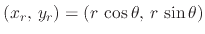. It can be observed that the choice ofaffects the height of the Lorentzian distribution, thereby affecting the height of the desired nanodot. Therefore, an additional contribution, dependent on, is needed in the equation for the height generated by each particle. This contribution is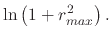(133)

The resulting nanodot cross section, shown in Figure 3.23 matches the ideal one-dimensional Lorentzian distribution. This method allows the generation of nanodots, such as the one shown in Figure 3.24, which follow a Lorentzian distribution, as desired for the AFM oxidation simulator.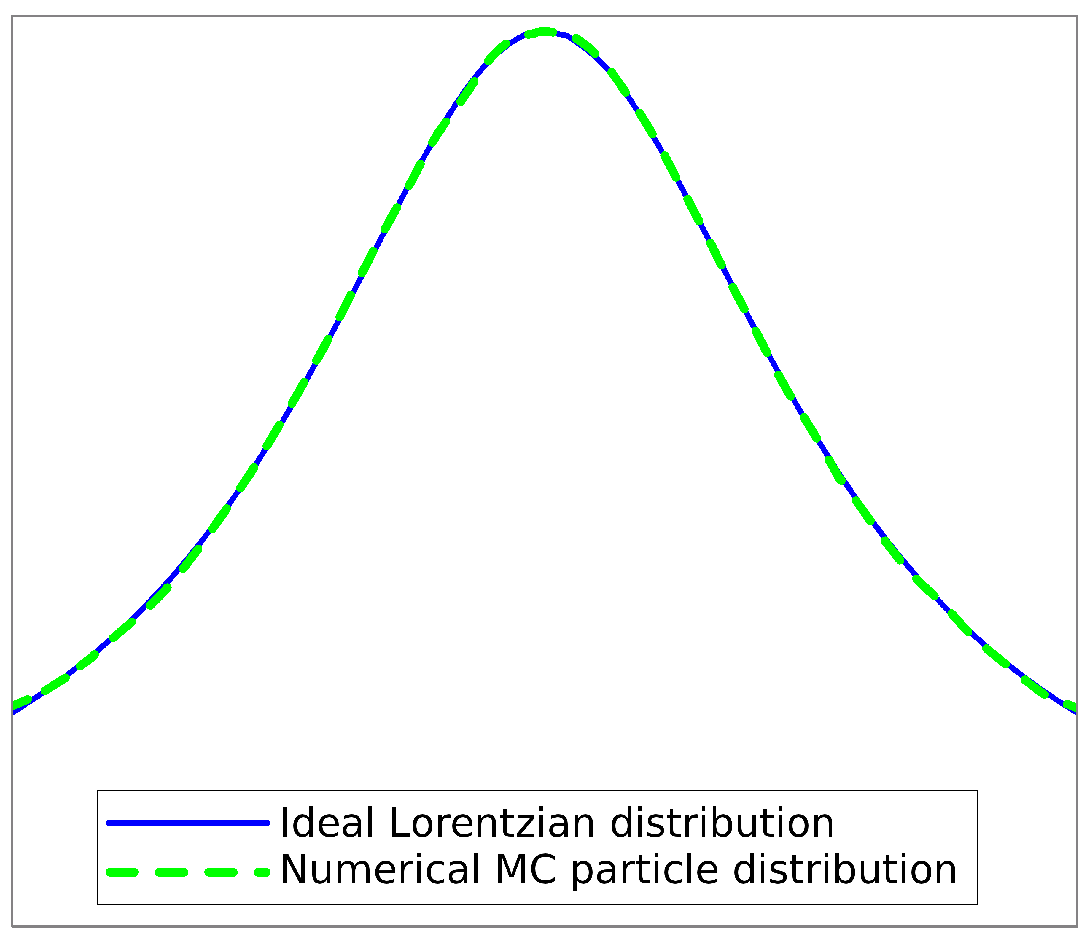Figure 3.24: The vertical dimension has been scaled by 20 for better visualization. (a) NCM nanodot generated using a Lorentzian distribution of particles and (b) Diagonal cross-section of the nanodot
from Figure 3.24a.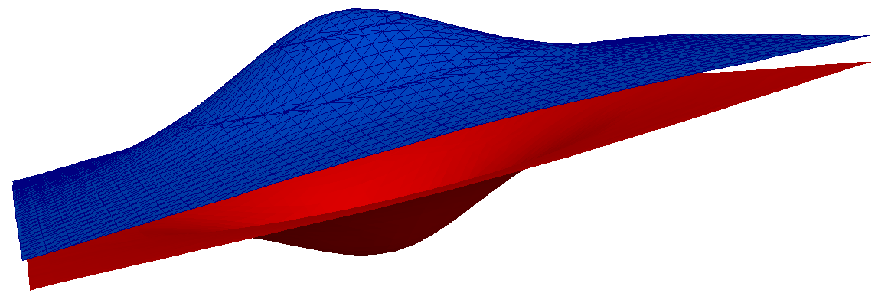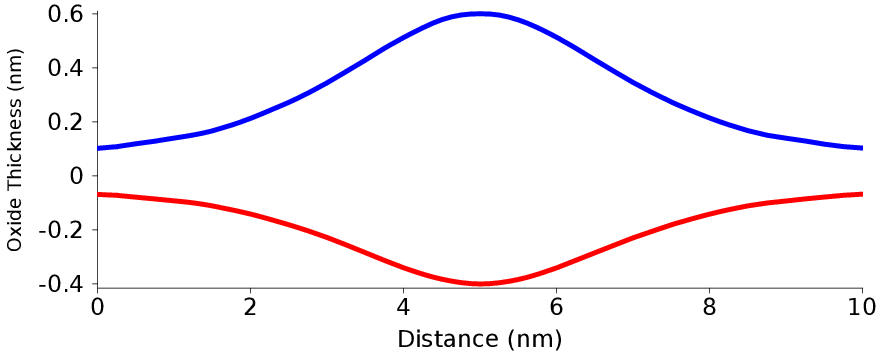(a) NCM Lorentzian nanodot. (b) Cross-section.

The expression for the quantile function in (3.61) suggests a potential connection to the Marsaglia polar method. A Lorentzian distribution can be generated using a similar procedure. The first step is, once again, the generation of an evenly distributed random location in two dimensions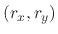within a circle with radius. The Lorentzian distributed coordinates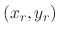can then be expressed as: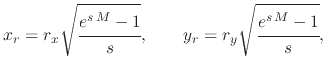(134)

where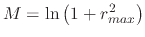.

### 3.4.3.3 One-Dimensional Surface Charge Density Particle Distribution

SCD charge density on the silicon wafer is advantageous since a nanodot can then be generated which directly follows the applied electric field, a function of the applied voltage at the AFM needle. When performing nanodot oxidation simulations using an AFM for a two-dimensional model, a one-dimensional particle distribution is required. The equation (3.37), for a SCD distribution of a hemispherical needle tip can be re-written in a one-dimensional form: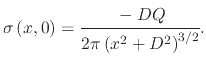(135)

(3.64) can then be used to generate a one-dimensional PDF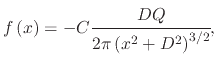(136)

whereis the normalization constant.is found by integrating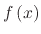over the entire simulation domain and equating it to unity: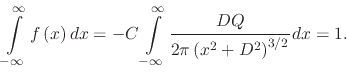(137)

By solving (3.66), the normalization constantis found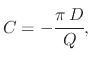(138)

which is then substituted into (3.65) to form the normalized PDF for a one-dimensional SCD distribution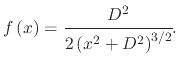(139)

The next step is finding the CPD function, derived by integrating the normalized PDF,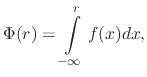(140)

whereis the SCD distributed radius. Because of the symmetry of the SCD distribution on either side of the charged particle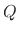, generating a CPD distributed radius becomes easier, when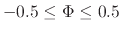. Therefore, we set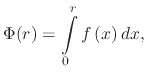(141)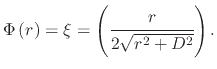(142)

Setting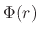equal to an evenly distributed random number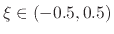and inverting (3.71) allows us to obtain the SCD quantile function required for particle generation: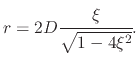(143)

Therefore, in order to generate particles obeying the SCD distribution along the silicon wafer surface, each particle must be generated using (3.72), whereis an evenly distributed random number,.

### 3.4.3.4 Two-Dimensional Surface Charge Density Particle Distribution

When working with a three-dimensional model for AFM oxidation of nanodots, a two-dimensional particle distribution is required. The analysis is similar to the one-dimensional model presented in the previous section. The derivation of the quantile function is performed using polar coordinates for simplicity and for easier generation of a final radial distribution of particles. For polar coordinates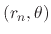it is important to note that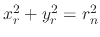, and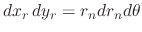. This was also discussed when deriving the Lorentzian distribution. The two-dimensional PDF, in polar coordinates, derived from (3.37) is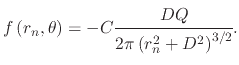(144)

The normalization constantis once again found by integrating the PDF over the entire simulation range and equating it to one: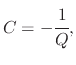(145)

then the normalized two-dimensional PDF in polar coordinates becomes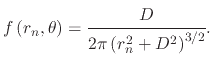(146)

The CPD is found by integrating the normalized PDF over the simulation area. The angular component results in a value of 2, while the radial component is found by first finding the radius-dependent CPD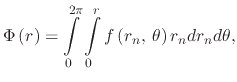(147)

which equates to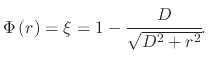(148)

The quantile function for the two-dimensional SCD distribution is found by inverting the CPD function to obtain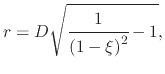(149)

whereis an evenly distributed random number,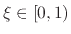. The angular component of the distribution is obtained in the same manner as the radial component for the Lorentzian distribution. An evenly distributed angle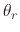between 0 and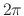is found and the final Cartesian location for each particle is given by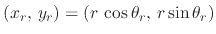. The normalized cross-section of a nanodot generated using this distribution is compared to the normalized SCD from (3.64) in Figure 3.25.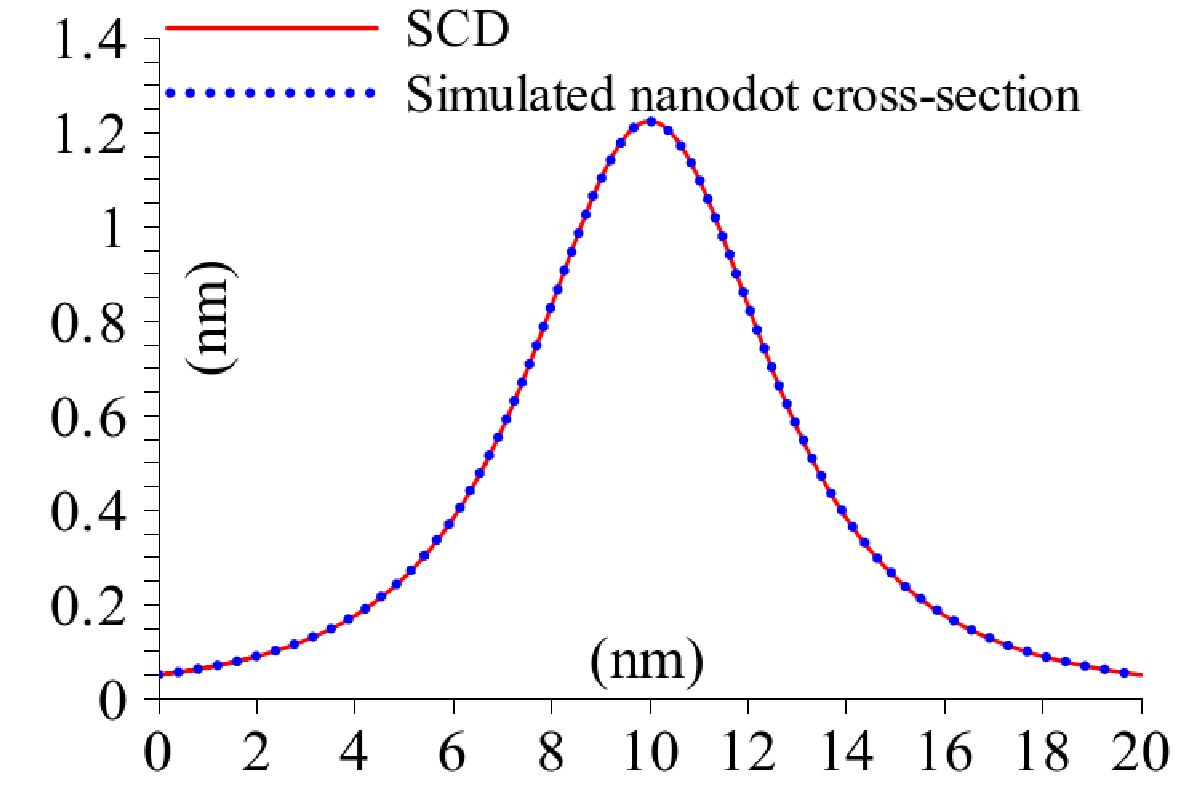### 3.4.3.5 Modeling Nanodots with Additional Point Charges

As previously mentioned, a rough needle tip must be modeled using a ring of charges at a given height above the silicon surface. The SCD distribution is found using the method of image charges and summing the effects of each individual charge which makes up the charged ring, resulting in the SCD distribution shown in (3.38).

The SCD in (3.38) does not allow for a straight-forward derivation of a random distribution, such as the one shown in (3.72) and (3.78). Therefore, the MC rejection technique, or the accept-reject algorithm must be applied, whereby a test point is generated on the entire simulation domain using an even distribution,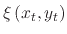. An additional evenly distributed number between zero and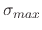from (3.39) is generated and, if this number is below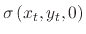, a particle is generated at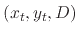. Otherwise, the locationis ignored and a new test point is generated. This procedure is repeated until a sufficient number of particles is kept in order to generate a nanodot topography.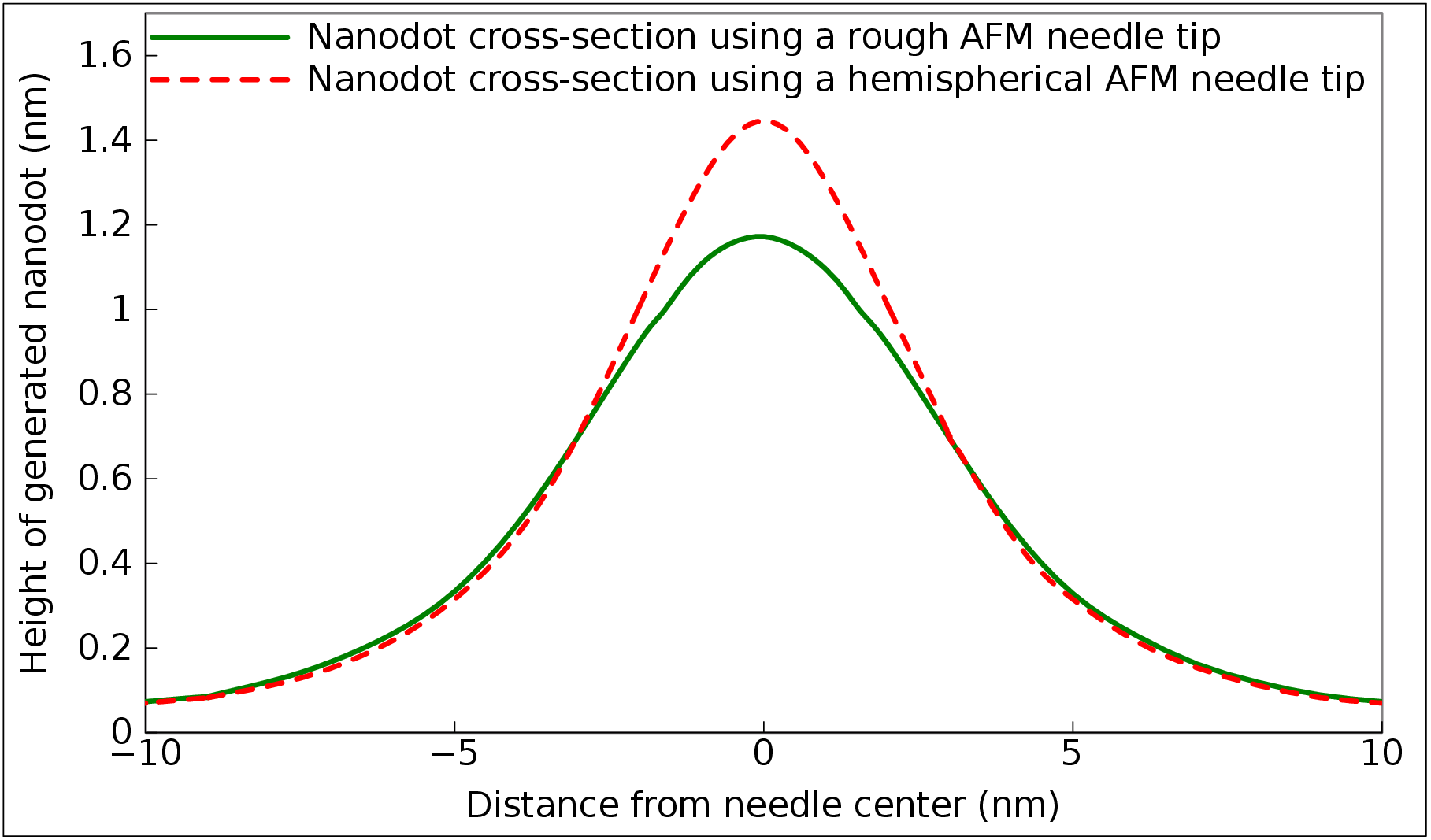It is evident that a blunt needle tip will result in a blunt nanodot formation with a slight increase in lateral spreading. The effective height of a nanodot when using a rough AFM needle tip versus a hemispherical AFM needle tip is shown in Figure 3.26. It is visible that the height at the middle of the nanodot is lower for a rough needle tip, as the electric field is spread laterally.

L. Filipovic: Topography Simulation of Novel Processing Techniques